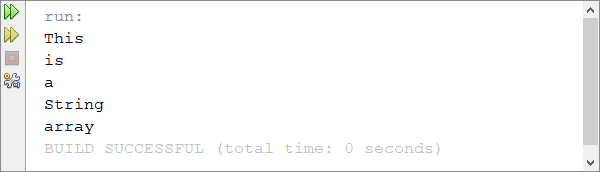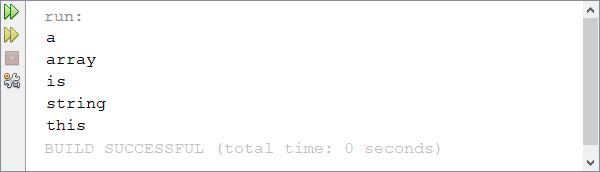Home and Learn: Games Programming Course

# Arrays and Strings

You can place strings of text into your Java arrays. This is done in the same way as for integers:

String[ ] aryString = new String ;

aryString = "This";
aryString = "is";
aryString = "a";
aryString = "string";
aryString = "array";

The code above sets up a string array with 5 positions. Text is then assigned to each position in the array.

Here's a loop that goes round all the positions in the array, printing out whatever is at each position:

int i;
for ( i=0; i < aryString.length; i++ ) {
System.out.println( aryString[i] );
}

The loop goes round and round while the value in the variable called i is less than the length of the array called aryString.

When the above programme is run, the Output window will look like this:You can perform a sort on string arrays, just like you can with integers. But the sort is an alphabetical ascending one, meaning that "aa" will come first over "ab". However, Java uses Unicode characters to compare one letter in your string to another. This means that uppercase letter will come before lowercase ones. Try the following code:When the programme is run, the Output window will display the following:Although we've sorted the array, the word "This" comes first. If this were an alphabetical sort, you'd expect the word "a" to come first." And it does if all the letters are lowercase. In your programming code, change the capital "T" of "This" to a lowercase "t". Now run your programme again. The Output window will now display the following:As you can see, the word "this" is now at the bottom. We'll have a closer look at strings in the next section, so don't worry too much about them now. Instead, try these exercises.

Exercise G
Set up an array to hold the following values, and in this order: 23, 6, 47, 35, 2, 14. Write a programme to get the average of all 6 numbers. (You can use integers for this exercise, which will round down your answer.)

Exercise H
Using the above values, have your programme print out the highest number in the array.

Exercise I
Using the same array above, have your programme print out only the odd numbers.